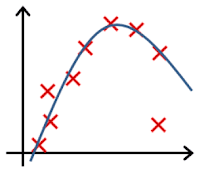# ▸ Regularization :

1. You are training a classification model with logistic regression. Which of the following statements are true? Check all that apply.
• Introducing regularization to the model always results in equal or better performance on the training set.
• Introducing regularization to the model always results in equal or better performance on examples not in the training set.
• Adding a new feature to the model always results in equal or better performance on the training set.
• Adding many new features to the model helps prevent overfitting on the training set.

1. Suppose you ran logistic regression twice, once with , and once with . One of the times, you got parameters , and the other time you got . However, you forgot which value of corresponds to which value of . Which one do you think corresponds to ?
• When is set to 1, We use regularization to penalize large value of . Thus, the parameter, , obtained will in general have smaller values.

1. Suppose you ran logistic regression twice, once with , and once with . One of the times, you got parameters , and the other time you got . However, you forgot which value of corresponds to which value of . Which one do you think corresponds to ?
• When is set to 1, We use regularization to penalize large value of . Thus, the parameter, , obtained will in general have smaller values.

1. Which of the following statements about regularization are true? Check all that apply.
• Using a very large value of hurt the performance of your hypothesis; the only reason we do not set to be too large is to avoid numerical problems.
• Because logistic regression outputs values , its range of output values can only be “shrunk” slightly by regularization anyway, so regularization is generally not helpful for it.
• Consider a classification problem. Adding regularization may cause your classifier to incorrectly classify some training examples (which it had correctly classified when not using regularization, i.e. when λ = 0).
• Using too large a value of λ can cause your hypothesis to overfit the data; this can be avoided by reducing λ.

1. Which of the following statements about regularization are true? Check all that apply.
• Using a very large value of hurt the performance of your hypothesis; the only reason we do not set to be too large is to avoid numerical problems.
• Because logistic regression outputs values , its range of output values can only be “shrunk” slightly by regularization anyway, so regularization is generally not helpful for it.
• Because regularization causes J(θ) to no longer be convex, gradient descent may
not always converge to the global minimum (when λ > 0, and when using an
appropriate learning rate α).
• Using too large a value of λ can cause your hypothesis to underfit the data; this can be avoided by reducing λ.

1. In which one of the following figures do you think the hypothesis has overfit the training set?
• Figure:• Figure:• Figure:• Figure:### Check-out our free tutorials on IOT (Internet of Things):

1. In which one of the following figures do you think the hypothesis has underfit the training set?
• Figure:• Figure:• Figure:• Figure:&
Click here to see more codes for Raspberry Pi 3 and similar Family.
&
Click here to see more codes for NodeMCU ESP8266 and similar Family.
&
Click here to see more codes for Arduino Mega (ATMega 2560) and similar Family.

Feel free to ask doubts in the comment section. I will try my best to answer it.
If you find this helpful by any mean like, comment and share the post.
This is the simplest way to encourage me to keep doing such work.

Thanks & Regards,
- APDaga DumpBox

1.Why 1. cannot be D)? I could not see any difference between example and training set.

1.I mean alternative B (instead of D)

2.3.Adding many new features will overfit the data instead of preventing overfitting

4.@Unknown True. Agreed.
That's the reason Why D is the wrong Answer for Q1.

5.Hi,
Yeah you are right, Adding many new features can cause overfitting and regularization such as L1 and L2 are actually pushing effects of some features close to zero. I am not sure why its not considered as correct answer in this quiz

2.Hello,
In question one, why B is not selected?
as regularisation generalizes for new examples.

1.Because option B is not correct.
Reason: Regularization does not "always" garantee the better result on new examples.